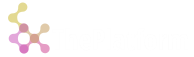🔍

# Platform

Platform uses work stealing as a task scheduling strategy and consists of three main components: `Runtime, `Scheduler, `Task.

In a work stealing runtime, each scheduler has a queue of tasks to perform. Each task consists of a series of instructions to be executed sequentially, but in the course of its execution, a task may also spawn new task that can feasibly be executed in parallel with the rest of its work. These new tasks are initially put on the queue of the scheduler executing the current (parent) task. When a scheduler runs out of work, it looks at the queues of other schedulers and "steals" their tasks. In effect, work stealing distributes the scheduling work over idle schedulers, and as long as all schedulers have work to do, no scheduling overhead occurs.

## Runtime

The purpose of Runtime is correct initialization and shutdown of workers' threads and schedulers. Also, it manages bootstrap or injector scheduler for creating root task.

``````extern crate rt;
use rt::runtime::{Runtime, spawn};

fn main() {
let num_cores = 2;
let stack_size = 1024;
Runtime::new(num_cores, stack_size).run(|| {
let hello = spawn(|| {
print!("Hello ");
});
hello.join().unwrap();
println!("world!");
});
}
``````

Usually, bootstrap scheduler is used as a dedicated processor to block operations (termіnal, files or network processing).

## Scheduler

Scheduler implements work stealing algorithm, manages tasks execution and rescheduling them if needed (more on this later). Under the hood uses Chase-Lev deque as a tasks queue.Usually, not directly accessible for an end-user.

Tasks are implemented via stackful coroutine. They can be rescheduled after explicit `this::yield_task()` call or implicitly on bounded channel operations:

``````let (s, r) = channel(2);// two element channel

s.push(41);
s.push(42);
s.push(43); //wait until r.pop() 43

runtime::spawn(|| {
let v = r.pop();
assert_eq!(41, v);
let v = r.pop();
assert_eq!(42, v);
let v = r.pop();
assert_eq!(43, v);

let v = r.pop(); // yield from task until next s.push(..)
});
``````

## Fork-Join Parallelism

Using `Runtime, `Scheduler and `Task is enough to implement fork-join parallelism. We can build acyclic DAG of computation using spawn (fork) and join (join) primitives.

## Channels and Pi-Calculus

Channels are a typed conduit through which you can send and receive vаlues to/from other tasks. Vаlues can be channels themselves as well (Pi-Calculus feature).

We have implemented several types of channel:

## Bounded single produser-single consumer

``````use sync::channel::channel;

let (s, r) = channel(8);
s.push(42);

runtime::spawn(|| {
let v = r.pop();
assert_eq!(42, v);
});
``````

## Unbounded multiple producers-single consumer

``````use sync::mpsc::channel;

let (s, r) = channel(); // no capacity. unbounded!

let s1 = s.clone();
runtime::spawn(|| {
s1.push(41);
});

let s2 = s.clone();
runtime::spawn(|| {
s2.push(42);
});

let v1 = r.pop();
let v2 = r.pop();

println!("[{}, {}]", v1, v2); //"[41, 42] or [42, 41]"
``````

## Publisher-Consumers

``````use queues::publisher::Publisher;

let mut publisher: Publisher<u32> = Publisher::with_capacity(8);

//write by 4 events at the same time
match publisher.next_n(4) {
Some(vs) => {
vs = 0;
vs = 1;
vs = 2;
vs = 3;
publisher.commit();
}
None => {} // not enough space for write
}

let subscriber = publisher.subscribe();
runtime::spawn(|| {
loop {
match subscriber.recv_n(4) {
Some(vs) => {
subscriber.commit();
assert_eq!([0, 1, 2, 4], vs);
}
None => {this::yield_task();} // no events
}
}
});

``````